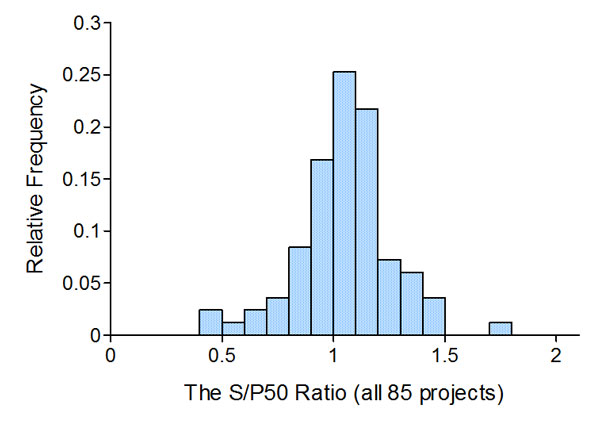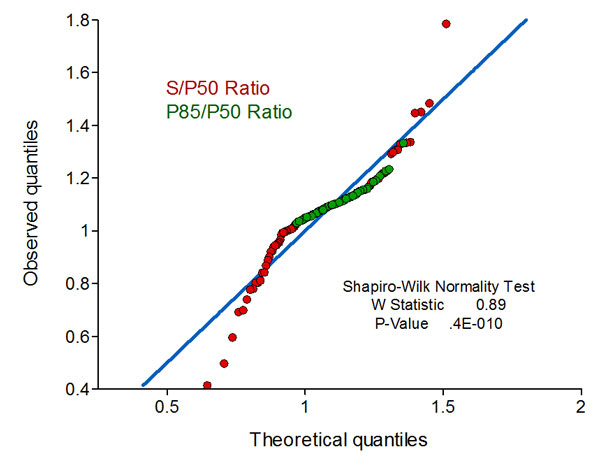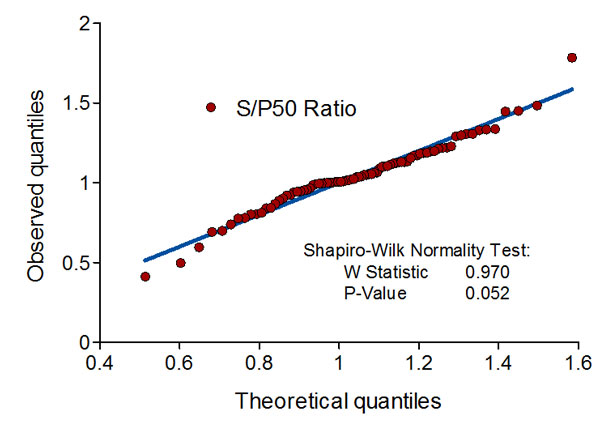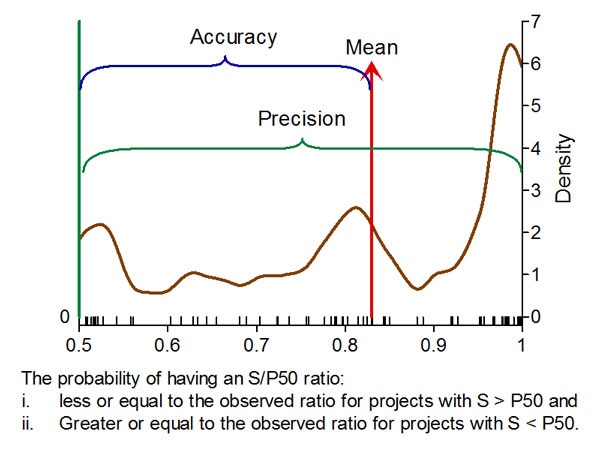# The Estimated Project Cost Distributions and the Final Project Cost

This entry is part 2 of 2 in the series The Norwegian Governmental Project Risk Assessment Scheme

Everybody believes in the exponential law of errors: the experimenters, because they think it can be proved by mathematics; and the mathematicians, because they believe it has been established by observation. (Whittaker& Robinson, 1967)

The growing use of Cost Risk Assessment models in public projects has raised some public concerns about its costs and the models ability to reduce cost overruns and correctly predict the projects final cost. We have in this article shown that the models are neither reliable nor valid, by calculating the probabilities of the projects final costs. The final cost and their probabilities indicate that the cost distributions do not adequately represent the actual cost distributions.

#### Introduction

In the previous post we found that the project cost distributions applied in the uncertainty analysis for 85 Norwegian public works projects were symmetric – and that they could be represented by normal distributions. Their P85/P50 ratios also suggested that they might come from the same normal distribution, since a normal distribution seemed to fit all the observed ratios. The quantile-quantile graph (q-q graph) below depicts this:As the normality test shows, it is not exactly normal1, but near enough normal for all practical purposes2. This was not what we would have expected to find.

The question now is if the use of normal distributions representing the total project cost is a fruitful approach or not.

We will study this by looking at the S/P50 ratio that is the ratio between the final (actual) total project cost – S and the P50 cost estimate. But first we will take a look at the projects individual cost distributions.

#### The individual cost distributions

By using the fact that the individual project’s cost are normally distributed and by using the P50 and P85 percentiles we can estimate the mean and variance for all the projects’ the cost distributions (Cook, 2010).

In the graph below we have plotted the estimated relative cost distribution (cost/P50) for the projects with the smallest (light green) and the largest (dark green) variance. Between these curves lie the relative cost distributions for all the 85 projects.

Between the light green and the blue curve we find 72 (85%) of the projects. The area between the blue and the dark green curve contains 13 of the projects – the projects with the highest variance:The differences between the individual relative cost distributions are therefore small. Average standard deviation for all 85 projects is 0.1035 with a coefficient of variation of 48%. For the 72 projects the average standard deviation are 0.0882 with a coefficient of variation of 36%. This is consistent with what we could see from the regression of P85 on P50.

It is bewildering that a portfolio of so diverse projects can end up with such a small range of normal distributed cost.

#### The S/P50 ratio

A frequency graph of the 85 observed ratios (S/P50) shows a pretty much symmetric distribution, with a pronounced peak. It is slightly positively skewed, with a mean of 1.05, a maximum value of 1.79, a minimum value of 0.41 and a coefficient of variation of 20.3%:At first glance this seems as a reasonable result; even if the spread is large, given that the project’s total cost has normal distributions.

If the estimated cost distribution(s) gives a good representation of the underlying cost distribution, then – S – should also belong to that distribution. Have in mind that the only value we know with certainty to belong to the underlying cost distribution is – S, i.e. the final total project cost.

It is there for of interest to find out if the S/P50 ratio(s) are consistent with the estimated distributions. We will try to investigate this by different routes, first by calculating at what probability the deviation of S from P50 occurred.

What we need to find is, for each of the 85 projects, the probability of having had a final cost ratio (S/P50):

i.    less or equal to the observed ratio for projects with S > P50 and
ii.   Greater or equal to the observed ratio for projects with S < P50.

The graph below depicts this. The maroon circles give the final cost ratio (S/P50) and their probabilities:A frequency graph of these probabilities should give a graph with a right tail, with most of the projects close to the 0.5 fractile (the median or P50 value), tapering off to the right as we move to higher fractiles.

We would thus anticipate that most projects have been finished at or close to the quality assurance schemes median value i.e., having had a probability of 0.5 for having had this or a lower (higher) value as final cost ratio, and that only a few would have significant deviations from this.

We will certainly not expect many of the final cost ratio probabilities above the 0.85 percentile (P85).

The final cost probability frequency graph will thus give us some of the completing information needed to assess the soundness of using methods and simulation techniques ending up with symmetric project cost distributions.

#### Final project cost ratio probability

The result is given in the graph below, where the red bars indicates projects that with probabilities of 85% or more should have had lower (or higher) final cost ratios:The result is far from what we expected: the projects probabilities are not concentrated at or close to 0.5 and the frequency graph is not tapering off to the right. On the contrary, the frequency of projects increases as we move to higher probabilities for the S/P50 ratios, and the highest frequency is for projects that with high probability should have had a much less or a much higher final cost:

1. The final project cost ratio probabilities have a mean of 0.83, a median at 0.84 and a coefficient of variation of 21%.
2. Of the 85 projects, 51 % have final cost ratios that had a probability of 84% or less of being lower (or higher) and 49% have final cost ratios that had a probability of 85% or more of being lower (higher).

Almost fifty percent of the projects have thus been seriously under or over budgeted or have had large cost over- or underruns – according to the cost distributions established by the QA2 process.

The cumulative frequency distribution below gives a more detailed description:It is difficult to say in what range the probability for the S/P85 ratio should have been for considering the estimated cost distributions to be “acceptable”. If the answer is “inside the quartile range”, then only 30% of the projects final cost forecasts can be regarded as acceptable.

#### The assumption of normally distributed total project costs

Based on the close relation between the P50 and P85 percentiles it is tempting to conclude that most if not all projects has had the same cost estimation validation process; using the same family of cost distributions, with the same shape parameter and assuming independent cost elements – ending up with a near normal or normal distribution for the projects total cost. I.e. all the P85/50 ratios belong to the same distribution.

If this is the case, then also the projects final costs ratios should also belong to the same distribution. In the q-q graph below, we have added the S/P50 ratios (red) to the P85/P50 ratios (green) from the first q-q graph. If both ratios are randomly drawn from the same distribution, they should all fall close onto the blue identity line:The ratios are clearly not normaly distributed; the S/P50 ratios ends mostly up in both tails and the shape of the plotted ratios now indicates a distribution with heavy tails or may be with bimodality. The two ratios is hence most likely not from the same distribution.
A q-q graphn with only the S/P50 ratios shows however that they might be normaly distributed, but have been taken from a different distribution than the P85/P50 ratios:The S/P50 ratios are clearly normaly distributed as they fall very close onto the identity line. The plotted ratios also indicates a little lighter tails than the corresponding theoretical distribution.

That the two sets of ratios so clearly are different is not surprising, since the S/P50 ratios have a coeficient of variation of 20% while the same metric is 4.6% for the P85/P50 ratios3 .

Since we want the S/P50 ratio to be as close to one as possible, we can regard the distribution of the S/P50 ratios as the QA2’s error distribution.This brings us to the question of the reliability and validity of the QA2 “certified” cost risk assessment model.

#### Reliability and Validity

The first that needs to be answered is then the certified model’s reliability in producing consistent results and second if the cost model really measures what we want to be measured.

1. We will try to answer this by using the S/P50 probabilities defined above to depict:
The precision4  of the forecasted costs distributions by the variance of the S/P50 probabilities, and
2. The accuracy (or trueness) of the forecasts, or the closeness of the mean of the probabilities for the S/P50 ratio to the forecasts median value – 0.5.A visual inspection of the graph gives an impression of both low precision and low accuracy:

• the probabilities have a coefficient of variation of 21% and a very high density of final project costs ending up in the cost distributions tail ends, and
• the mean of the probabilities is 0.83 giving a very low accuracy of the forecasts.

The conclusion then must be that the cost models (s) are neither reliable nor valid:

####Summary

We have in these two articles shown that the implementation of the QA2 scheme in Norway ends up with normally distributed project costs.

i.    The final cost ratios (S/P50) and their probabilities indicate that the cost distributions do not adequately represent the actual distributions.
ii.    The model (s) is neither reliable nor valid.
iii.    We believe that this is due to the choice of risk models and technique and not to the actual risk assessment work.
iv.    The only way to resolve this is to use proper Monte Carlo simulation models and techniques

#### Final Words

Our work reported in these two posts have been done out of pure curiosity after watching the program “Brennpunkt”. The data used have been taken from the program’s documentation.  Based on the results, we feel that our work should be replicated by the Department of Finance and with data from the original sources, to weed out possible errors.

It should certainly be worth the effort:

i.    The 85 project here, amounts to NOK 221.005 million with
ii.    NOK 28.012 million in total deviation5  from the P50 value
iii.    NOK 19.495 million have unnecessary been held in reserve6  and
iv.    The overruns7  have been NOK 20.539 million
v.    That is, nearly every fifth “krone” of the projects budgets has been “miss” allocated
vi.    And there are many more projects to come.

#### References

Cook, J.D., (2010). Determining distribution parameters from quantiles.
http://biostats.bepress.com/mdandersonbiostat/paper55

Whittaker, E. T. and Robinson, G. (1967), Normal Frequency Distribution. Ch. 8 in The Calculus of Observations: A Treatise on Numerical Mathematics, 4th ed. New York: Dover, pp. 164-208, 1967. p. 179.

Series Navigation<< The implementation of the Norwegian Governmental Project Risk Assessment scheme
1. As the graph shows is the distribution slightly skewed to the right []
2. The corresponding linear regression gives a value of 0.9540 for the coefficient of determination (R). []
3. The S/P50 ratios have a mean of 1.0486 and a standard deviation of 0.2133. The same metrics for the P85/P50 ratios is 1.1069 and 0.0511. []
4. ISO 5725-Accuracy of Measurement Methods and Results. []
5. The sum of all deviations from the P50 values. []
6. The P85 amount less the final project cost > zero. []
7. The final project cost less the P50 amount > zero []